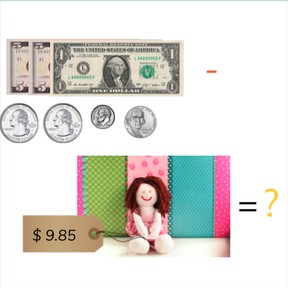Subtraction with decimal numbers with 1 or 2 decimal places

# Subtraction with decimal numbers with 1 or 2 decimal places8,000 schools use Gynzy92,000 teachers use Gynzy1,600,000 students use Gynzy

## General

Students learn to subtract decimal numbers with 1 or 2 decimal places. They also learn to solve story problems involving decimal numbers.

5.NBT.B.7

## Relevance

It is important that students are able to subtract decimal numbers with 1 or 2 decimal places, so they can determine how much money they have left after purchasing something.

## Introduction

Have students practice counting backward with decimal numbers with 1 or 2 decimal places. Using the snake students are asked to solve subtraction problems with one decimal place. Students are then asked to solve subtraction problems on the rope with subtraction problems with two decimal places. Spread the starting numbers between the groups. IF you have more than 6 groups in class, you can come up with extra numbers. Example: Group 1 gets the number 60.9, They start at the tail of the snake and solve to the head. The subtraction problem becomes 60.9 - 0.3 = 60.6. Then they must subtract another 0.3. Continue until they reach the last square. Check the differences together.

## Development

Using the number line, explain to students how to subtract decimal numbers with 1 decimal place. Tell students that you will give them two methods they can use to subtract decimal numbers. For the first method, show that you leave the first number (the minuend)- 56.9 as a whole number, and that you split the second number (the subtrahend)- 31.2 into two parts, 31 and 0.2. Using the number line, first subtract the 31. Then take away the 0.2. For the second method, show that you split both numbers in the subtraction problem in to the number before the decimal point and the number after the decimal point. In the problem 56.9 - 31.2 the first subtraction problem becomes 56 - 31 and the second problem becomes 0.9 - 0.2. Solve both of these problems and then add the two together to find the difference. Have students solve the next subtraction problem with 1 decimal place individually or in pairs and ask them to explain their strategy. Next discuss how to subtract decimal numbers with 2 decimal places. Explain the problem 42.83 - 27.15 using both methods applied to subtraction with 1 decimal place. Emphasize that they may choose the method that they feel is best suited to the subtraction problem or the one that they find easiest or best to use. The next problems are story problems. Remind students of the steps they need to take to solve a story problem. They first need to determine what kind of math problem is presented, to determine which numbers are important for the problem, to say or write the problem, and then finally to solve the math problem. Solve a story problem together with the class and then ask students to solve the next story problem individually or in pairs. Have students say the steps out loud.

Check that students are able to subtract with decimal numbers with 1 or 2 decimal places by asking the following questions:
- What are the methods you can use to solve a subtraction problem with 1 or 2 decimal places?
- Which method do you like to use? Why?
- How do you solve a story problem?
- What are the steps to solve a story problem?

## Guided practice

Students are given abstract subtraction problems with 1 or 2 decimal places and are given decimal story problems to solve. The problems increase in difficulty.

## Closing

Check that students are able to explain their strategy for subtracting decimal numbers with 1 or 2 decimal places. Also make sure that students can name the steps they use to solve a story problem. Use the subtraction problems given to check that students are able to subtract decimal numbers with 1 or 2 decimal places individually. Emphasize that they can choose the method that they like best to solve the problems. To close the lesson, ask students to determine the subtraction problem which has the given number as its difference. Tell them to make sure that they have a number with the right number of decimal places.

## Teaching tips

Students who have difficulty subtracting decimal numbers with 1 or 2 decimal places can be supported by placing decimal numbers on the number line. Start with decimal numbers with 1 decimal place and have them take away 0.1 from the given number. Have them draw that step on the number line. Make sure to practice the step between 1 to 0.9, 10 to 9.9, 15 to 14.9, etc. When students are comfortable with 1 decimal place you can practice with 2 decimal places.

## Instruction materials

Optional: number line

### The online teaching platform for interactive whiteboards and displays in schools

• Save time building lessons

• Manage the classroom more efficiently

• Increase student engagement